## Modular Angle

Given a Modulus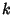in an Elliptic Integral, the modular angle is defined by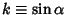. An Elliptic Integral is written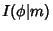when the Parameter is used,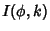when the Modulus is used, and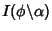when the modular angle is used.

See also Amplitude, Characteristic (Elliptic Integral), Elliptic Integral, Modulus (Elliptic Integral), Nome, Parameter

References

Abramowitz, M. and Stegun, C. A. (Eds.). Handbook of Mathematical Functions with Formulas, Graphs, and Mathematical Tables, 9th printing. New York: Dover, p. 590, 1972.